# Section 1.4. UWB Signals

### 1.4. UWB Signals

As defined by the FCC's First Report and Order, UWB signals must have bandwidths of greater than 500 MHz or a fractional bandwidth larger than 20 percent at all times of transmission . Fractional bandwidth is a factor used to classify signals as narrowband, wideband, or ultra-wideband and is defined by the ratio of bandwidth at 10 dB points to center frequency. Equation 1-4 shows this relationship.

 The 10 dB point represents the spectral power of a signal at 10 dB lower than its peak power.

Equation 1-4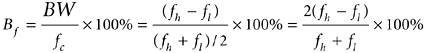where fh and f1 are the highest and lowest cutoff frequencies (at the 10 dB point) of a UWB pulse spectrum, respectively. A UWB signal can be any one of a variety of wideband signals, such as Gaussian, chirp, wavelet, or Hermite-based short-duration pulses. Figure 1-5 represents a Gaussian monocycle as an example of a UWB pulse in the time and frequency domains. The Gaussian monocycle is the first derivative of a Gaussian pulse and is given by

Equation 1-5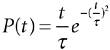##### Figure 1-5. A 500-picosecond Gaussian monocycle in (a) the time domain and (b) the frequency domain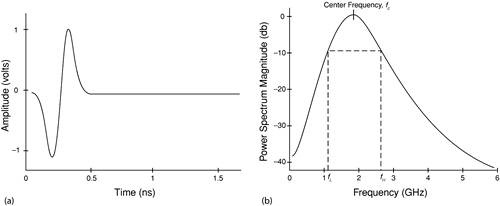where t represents time and t is a time decay constant that determines the temporal width of the pulse.

As shown in Figure 1-5, a 500-picosecond pulse generates a large bandwidth in the frequency domain with a center frequency of 2 GHz. In Figure 15b, the lowest and highest cutoff frequencies at 10 dB are approximately 1.2 GHz and 2.8 GHz, respectively, which lead to a fractional bandwidth of 80 percent; this is much larger than the minimum Bf required by the FCC: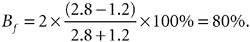Here is the classification of signals based on their fractional bandwidth:

 Narrowband Bf < 1% Wideband 1% < Bf < 20% Ultra-Wideband Bf > 20%

For example, 802.11 and Bluetooth have fractional bandwidths of 0.8 percent and 0.04 percent, respectively.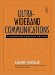Ultra-Wideband Communications: Fundamentals and Applications
ISBN: 0131463268
EAN: 2147483647
Year: 2005
Pages: 93

flylib.com © 2008-2017.
If you may any questions please contact us: flylib@qtcs.net